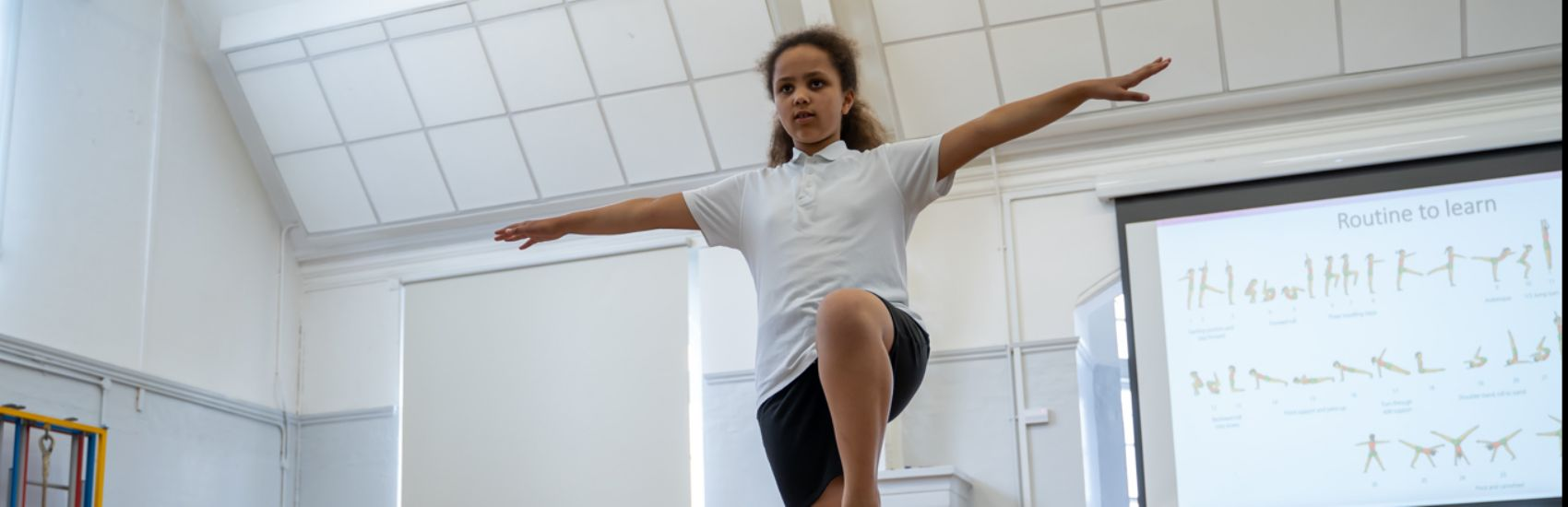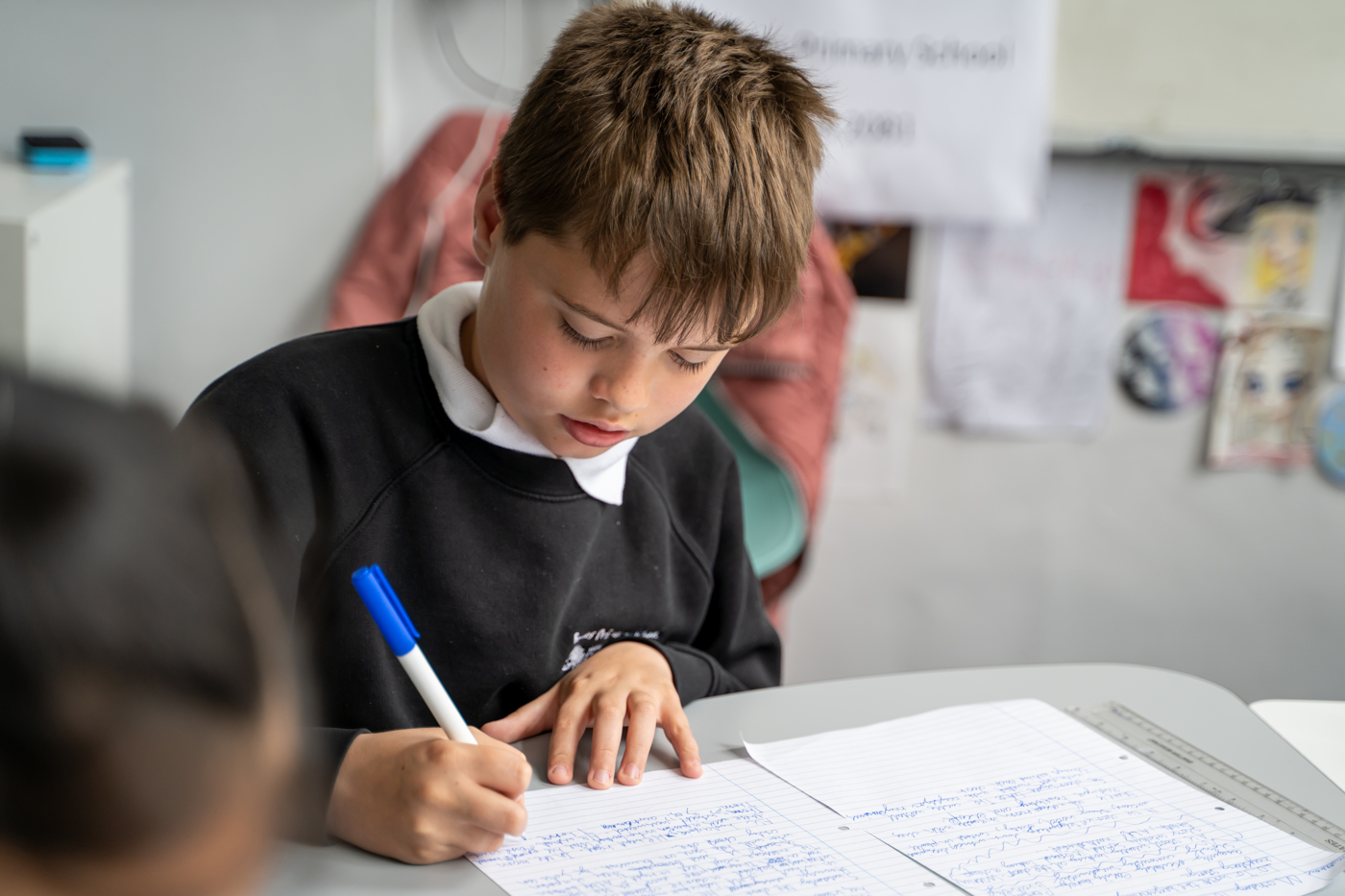''

# Mathematics

Mathematics National Curriculum for Year 6

Number - number and place value
The children will be taught to:
• read, write, order and compare numbers up to 10 000 000 and determine the value of each digit
• round any whole number to a required degree of accuracy
• use negative numbers in context, and calculate intervals across zero
• solve number and practical problems that involve all of the above.
Number - addition, subtraction, multiplication and division
The children will be taught to:
• multiply multi-digit numbers up to 4 digits by a two-digit whole number using the formal written method of long multiplication
• divide numbers up to 4 digits by a two-digit whole number using the formal written method of long division, and interpret remainders as whole number remainders, fractions, or by rounding, as appropriate for the context
• divide numbers up to 4 digits by a two-digit number using the formal written method of short division where appropriate, interpreting remainders according to the context
• perform mental calculations, including with mixed operations and large numbers
• identify common factors, common multiples and prime numbers
• use their knowledge of the order of operations to carry out calculations involving the four operations
• solve addition and subtraction multi-step problems in contexts, deciding which operations and methods to use and why
• solve problems involving addition, subtraction, multiplication and divsion
• use estimation to check answers to calculations and determine, in the context of a problem, an appropriate degree of accuracy.
Number - fractions (including decimals and percentages)
The children will be taught to:
• use common factors to simplify fractions; use common multiples to express fractions in the same denomination
• compare and order fractions, including fractions > 1
• add and subtract fractions with different denominators and mixed numbers, using the concept of equivalent fractions
• multiply simple pairs of proper fractions, writing the answer in its simplest form
• divide proper fractions by whole numbers
• associate a fraction with division and calculate decimal fraction equivalents [for example, 0.375] for a simple fraction
• identify the value of each digit in numbers given to three decimal places and multiply and divide numbers by 10, 100 and 1000 giving answers up to three decimal places
• multiply one-digit numbers with up to two decimal places by whole numbers
• use written division methods in cases where the answer has up to two decimal places
• solve problems which require answers to be rounded to specified degrees of accuracy
• recall and use equivalences between simple fractions, decimals and percentages, including in different contexts.
Ratio and proportion
The children will be taught to:
• solve problems involving the relative sizes of two quantities where missing values can be found by using integer multiplication and division facts
• solve problems involving the calculation of percentages [for example, of measures, and such as 15% of 360] and the use of percentages for comparison
• solve problems involving similar shapes where the scale factor is known or can be found
• solve problems involving unequal sharing and grouping using knowledge of fractions and multiples.
Algebra
The children will be taught to:
• use simple formulae
• generate and describe linear number sequences
• express missing number problems algebraically
• find pairs of numbers that satisfy an equation with two unknowns
• enumerate possibilities of combinations of two variables.
Measurement
The children will be taught to:
• solve problems involving the calculation and conversion of units of measure, using decimal notation up to three decimal places where appropriate
• use, read, write and convert between standard units, converting measurements of length, mass, volume and time from a smaller unit of measure to a larger unit, and vice versa, using decimal notation to up to three decimal places
• convert between miles and kilometres
• recognise that shapes with the same areas can have different perimeters and vice versa
• recognise when it is possible to use formulae for area and volume of shapes
• calculate the area of parallelograms and triangles
• calculate, estimate and compare volume of cubes and cuboids using standard units, including cubic centimetres (cm3) and cubic metres (m3), and extending to other units [for example, mm3 and km3].
Geometry - properties of shapes
The children will be taught to:
• draw 2-D shapes using given dimensions and angles
• recognise, describe and build simple 3-D shapes, including making nets
• compare and classify geometric shapes based on their properties and sizes and find unknown angles in any triangles, quadrilaterals, and regular polygons
• illustrate and name parts of circles, including radius, diameter and circumference and know that the diameter is twice the radius
• recognise angles where they meet at a point, are on a straight line, or are vertically opposite, and find missing angles.
Geometry - position and direction
The children will be taught to:
• describe positions on the full coordinate grid (all four quadrants)
• draw and translate simple shapes on the coordinate plane, and reflect them in the axes.
Statistics
The children will be taught to:
• interpret and construct pie charts and line graphs and use these to solve problems
• calculate and interpret the mean as an average.